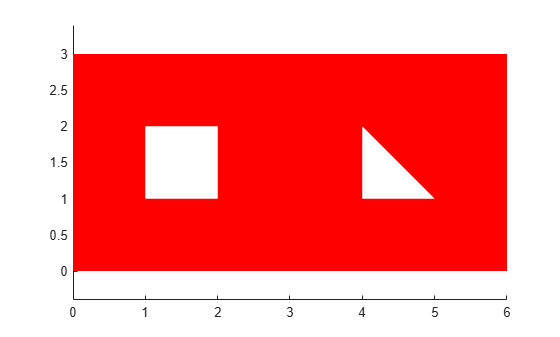# poly2fv

Convert polygon coordinates to patch faces and vertices

## Syntax

``[F,V] = poly2fv(x,y)``

## Description

example

````[F,V] = poly2fv(x,y)` converts the polygon coordinates defined by `x` and `y` into the triangular polygon regions defined by `V` and `F`, where `V` contains the vertices and `F` determines the vertices to connect.You can use the outputs of this function to display polygon regions by using the `patch` function.```

## Examples

collapse all

Specify the xy-coordinates of three polygons:

• A rectangle with vertices in a clockwise order.

• A square with vertices in a counterclockwise order.

• A triangle with vertices in a counterclockwise order.

```x1 = [0 0 6 6 0]; y1 = [0 3 3 0 0]; x2 = [1 2 2 1 1]; y2 = [1 1 2 2 1]; x3 = [4 5 4 4]; y3 = [1 1 2 1];```

Convert the coordinates to patch faces and vertices.

`[F,V] = poly2fv({x1,x2,x3},{y1,y2,y3});`

Display the patch.

```figure patch("Faces",F,"Vertices",V,"FaceColor","r","EdgeColor","none") axis equal```## Input Arguments

collapse all

x-coordinates of the polygon, specified as a numeric vector or a cell array of numeric vectors.

• Define one polygon by specifying a vector, such as ```[39 45 19 39]```.

• Define multiple polygons by using one of these options:

• Specify a vector and separate the polygons using `NaN` values, such as ```[37 46 31 20 37 NaN 45 49 35 32 45 NaN 35 40 42 35]```. The `NaN` values in `x` must correspond to the `NaN` values in `y`.

• Specify a cell array of vectors, such as ```{[37 46 31 20 37],[45 49 35 32 45],[35 40 42 35]}```. The size of the vector in each cell of `x` must match the size of the vector in the corresponding cell of `y`.

The size and type of `x` must match the size and type of `y`.

y-coordinates of the polygon, specified as a numeric vector or a cell array of numeric vectors.

• Define one polygon by specifying a vector, such as ```[-113 -49 -100 -113]```.

• Define multiple polygons by using one of these options:

• Specify a vector and separate the polygons using `NaN` values, such as ```[69 90 105 79 69 NaN 6 52 43 14 6 NaN 18 32 22 18]```. The `NaN` values in `y` must correspond to the `NaN` values in `x`.

• Specify a cell array of vectors, such as ```{[69 90 105 79 69],[6 52 43 14 6],[18 32 22 18]}```. The size of the vector in each cell of `y` must match the size of the vector in the corresponding cell of `x`.

The size and type of `y` must match the size and type of `x`.

## Output Arguments

collapse all

Polygon face definitions, returned as a three-column matrix that determines which vertices in `V` to connect. The `poly2fv` function creates triangular faces.

Data Types: `double`

Polygon vertices, returned as a two-column matrix. Each row of the matrix contains the xy-coordinates of a vertex.

Data Types: `double`

## Tips

Most Mapping Toolbox™ functions assume that clockwise-ordered vertices define external polygon edges and counterclockwise-ordered vertices define internal polygon edges. Although the `poly2fv` function ignores vertex order, you can ensure consistency with other functions by following this convention.

## Version History

Introduced before R2006a

expand all